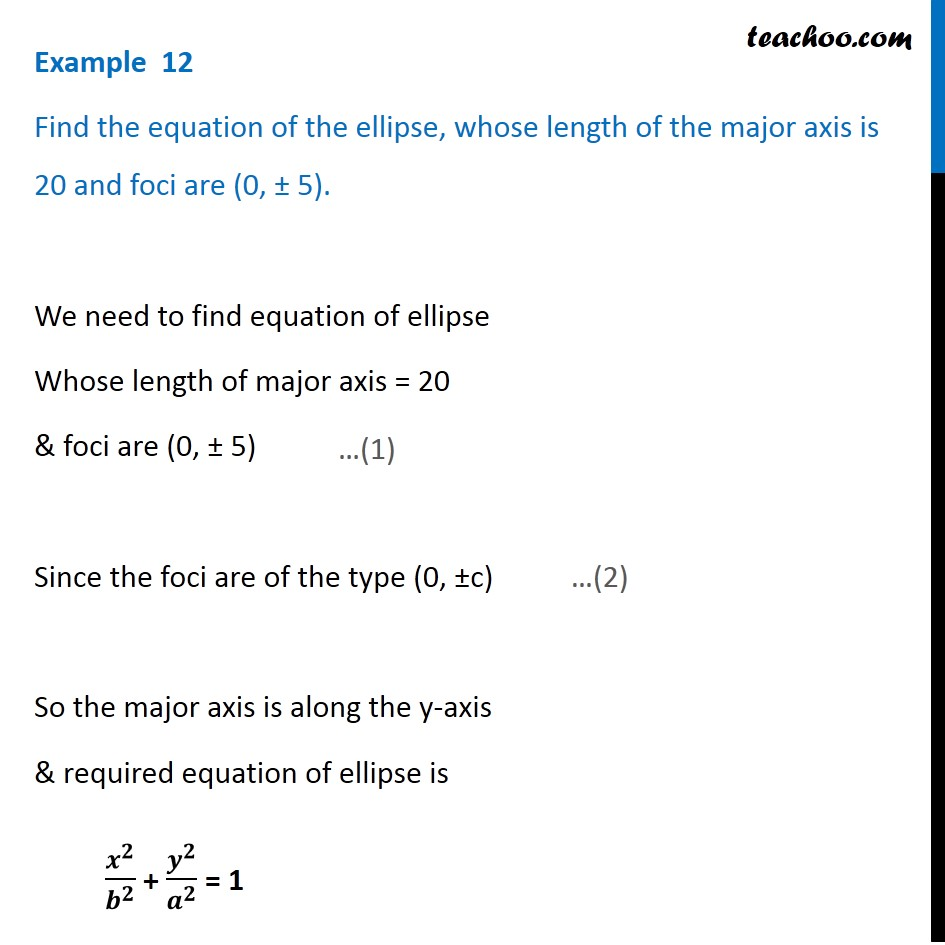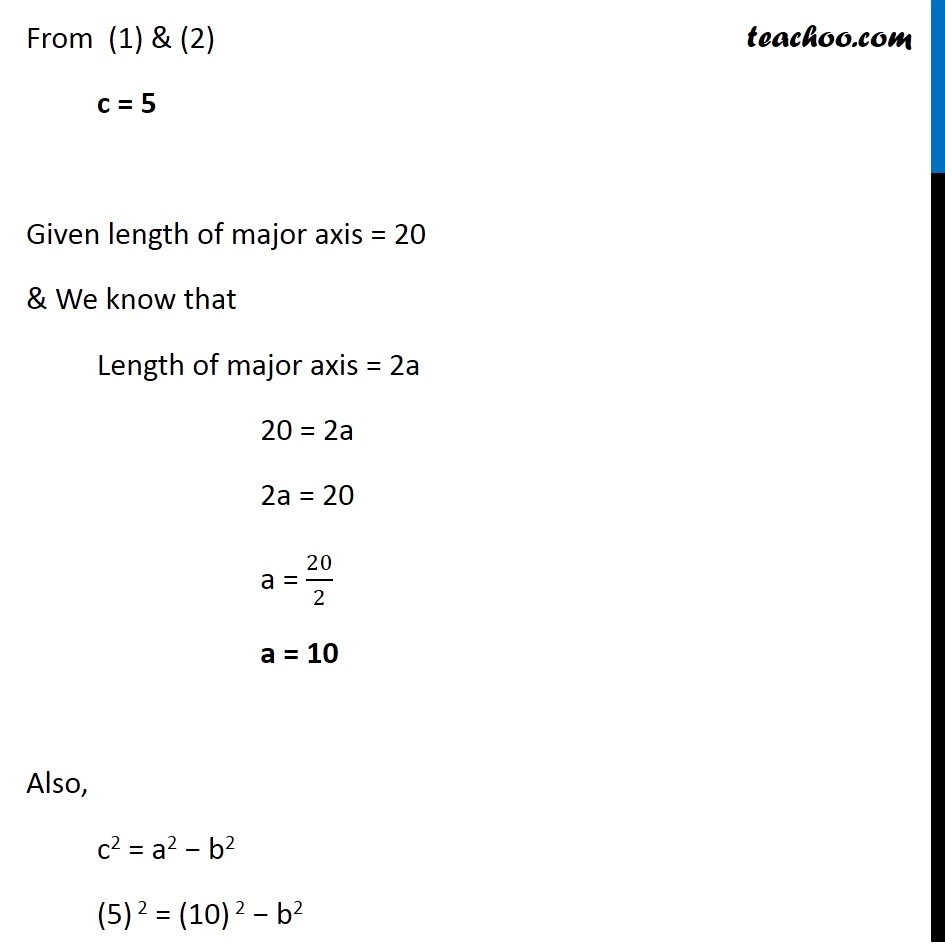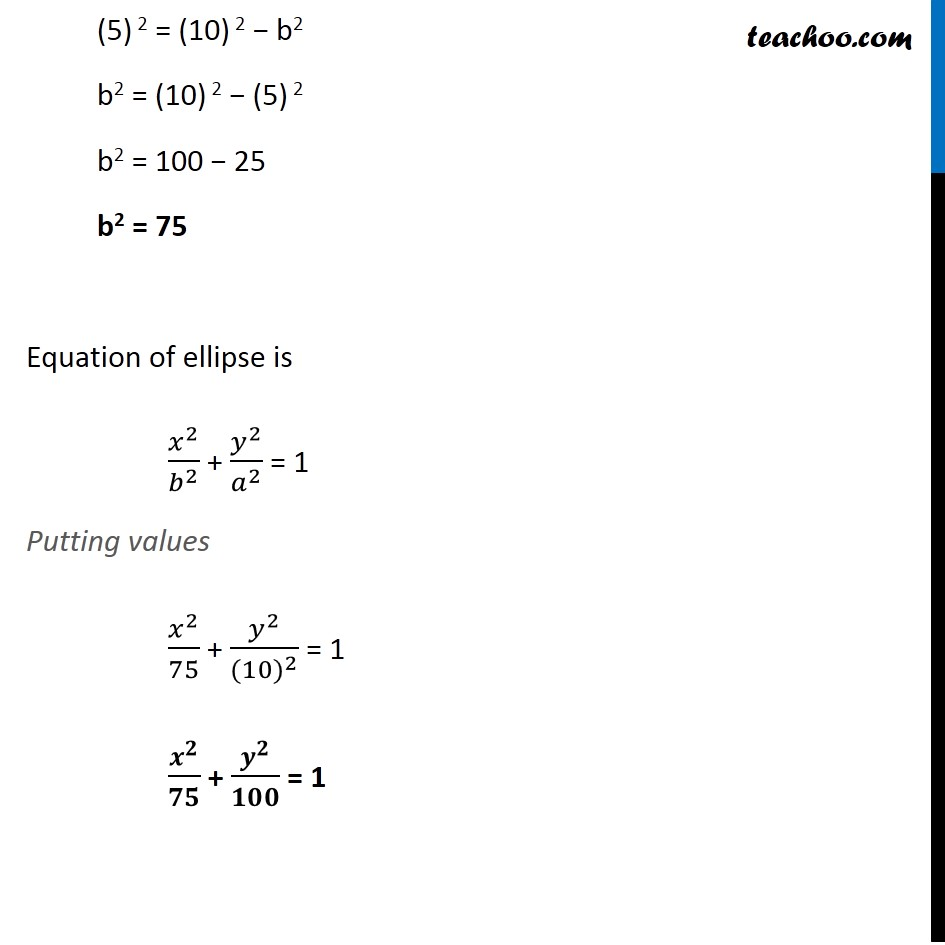Examples

Chapter 11 Class 11 Conic Sections
Serial order wiseMaths Crash Course - Live lectures + all videos + Real time Doubt solving!

### Transcript

Example 12 Find the equation of the ellipse, whose length of the major axis is 20 and foci are (0, ± 5). We need to find equation of ellipse Whose length of major axis = 20 & foci are (0, ± 5) Since the foci are of the type (0, ±c) So the major axis is along the y-axis & required equation of ellipse is 𝒙^𝟐/𝒃^𝟐 + 𝒚^𝟐/𝒂^𝟐 = 1 From (1) & (2) c = 5 Given length of major axis = 20 & We know that Length of major axis = 2a 20 = 2a 2a = 20 a = 20/2 a = 10 Also, c2 = a2 − b2 (5) 2 = (10) 2 − b2 (5) 2 = (10) 2 − b2 b2 = (10) 2 − (5) 2 b2 = 100 − 25 b2 = 75 Equation of ellipse is 𝑥^2/𝑏^2 + 𝑦^2/𝑎^2 = 1 Putting values 𝑥^2/75 + 𝑦^2/〖(10)〗^2 = 1 𝒙^𝟐/𝟕𝟓 + 𝒚^𝟐/𝟏𝟎𝟎 = 1